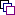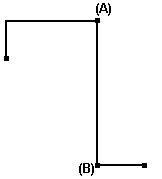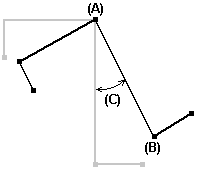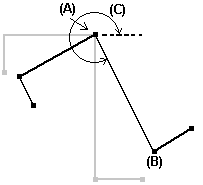# Rotate Ribbon - SmartSketch - Help

## SmartSketch Help

PPMProduct
SmartSketch
PPMCategory_custom
Help
Version_SSK
7.1(2011R1)Copy

Creates a copy of the element that you are rotating. If you do not want a copy of the rotated element, then clear this option.

Step Angle

Specifies the rotation step angle. The step angle specifies the increments, in degrees, that an element rotates from a temporary axis. The temporary axis is the line between the element's center of rotation (A) and the point that you rotate the element from (B).You can specify increments in the Step Angle box if you want to dynamically view the results of the rotation. For example, if the box is set to 30.0, the rotation is displayed in 30 degree increments. The default setting is 0 degrees so that the rotation is fully dynamic.

Rotation Angle

Defines the rotation angle. The rotation angle is the angle between the point that you rotate from and the point that you rotate to. In the following picture, the center of rotation (A) is shown with the point that you rotate the element from (B).The rotation angle (C) is the angle of change between the old and new positions of the rotated element. The value is always positive.Position Angle

Displays the angle between the horizontal axis through the center of rotation (A) and the point that you rotate from (B). Then, if you rotate the element, the position angle (C) changes to become the angle between the horizontal axis and the point that you rotate to. The position angle is always a positive value that is measured counter-clockwise from the axis.